# Bayes' rule: Functional form

Bayes’ rule generalizes to continuous functions, and states, “The posterior probability density is proportional to the likelihood function times the prior probability density.”

$$\mathbb P(H_x\mid e) \propto \mathcal L_e(H_x) \cdot \mathbb P(H_x)$$

## Example

Suppose we have a biased coin with an unknown bias $$b$$ between 0 and 1 of coming up heads on each individual coinflip. Since the bias $$b$$ is a continuous variable, we express our beliefs about the coin’s bias using a probability density function $$\mathbb P(b),$$ where $$\mathbb P(b)\cdot \mathrm{d}b$$ is the probability that $$b$$ is in the interval $$[b + \mathrm{d}b]$$ for $$\mathrm db$$ small. (Specifically, the probability that $$b$$ is in the interval $$[a, b]$$ is $$\int_a^b \mathbb P(b) \, \mathrm db.$$)

By hypothesis, we start out completely ignorant of the bias $$b,$$ meaning that all initial values for $$b$$ are equally likely. Thus, $$\mathbb P(b) = 1$$ for all values of $$b,$$ which means that $$\mathbb P(b)\, \mathrm db = \mathrm db$$ (e.g., the chance of $$b$$ being found in the interval from 0.72 to 0.76 is 0.04).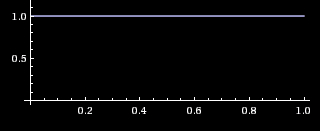We then flip the coin, and observe it to come up tails. This is our first piece of evidence. The likelihood $$\mathcal L_{t_1}(b)$$ of observation $$t_1$$ given bias $$b$$ is a continuous function of $$b$$, equal to 0.4 if $$b = 0.6,$$ 0.67 if $$b = 0.33,$$ and so on (because $$b$$ is the probability of heads and the observation was tails).

Graphing the likelihood function $$\mathcal L_{t_1}(b)$$ as it takes in the fixed evidence $$t_1$$ and ranges over variable $$b,$$ we obtain the straightforward graph $$\mathcal L_{t_1}(b) = 1 - b.$$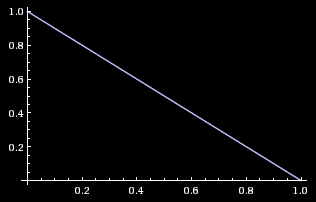If we multiply the likelihood function by the prior probability function as it ranges over $$b$$, we obtain a relative probability function on the posterior, $$\mathbb O(b\mid t_1) = \mathcal L_{t_1}(b) \cdot \mathbb P(b) = 1 - b,$$ which gives us the same graph again:But this can’t be our posterior probability function because it doesn’t integrate to 1. $$\int_0^1 (1 - b) \, \mathrm db = \frac{1}{2}.$$ (The area under a triangle is half the base times the height.) Normalizing this relative probability function will give us the posterior probability function:

$$\mathbb P(b \mid t_1) = \dfrac{\mathbb O(b \mid t_1)}{\int_0^1 \mathbb O(b \mid t_1) \, \mathrm db} = 2 \cdot (1 - f)$$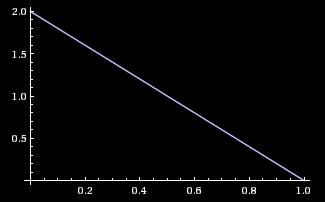The shapes are the same, and only the y-axis labels have changed to reflect the different heights of the pre-normalized and normalized function.Regraph these graphs with actual height changes

Suppose we now flip the coin another two times, and it comes up heads then tails. We’ll denote this piece of evidence $$h_2t_3.$$ Although these two coin tosses pull our beliefs about $$b$$ in opposite directions, they don’t cancel out — far from it! In fact, one value of $$b$$ (“the coin is always tails”) is completely eliminated by this evidence, and many extreme values of $$b$$ (“almost always heads” and “almost always tails”) are hit badly. That is, while the heads and the coins tails pull our beliefs in opposite directions, they don’t pull with the same strength on all possible values of $$b.$$

We multiply the old beliefby the additional pieces of evidence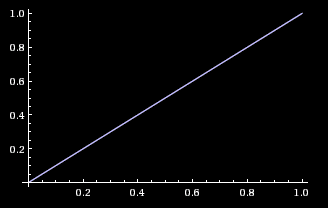andand obtain the posterior relative density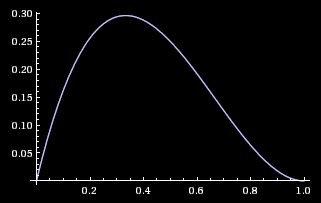which is proportional to the normalized posterior probability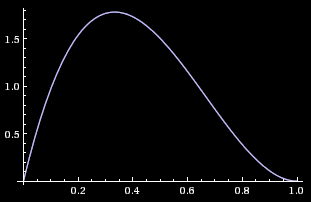Writing out the whole operation from scratch:

$$\mathbb P(b \mid t_1h_2t_3) = \frac{\mathcal L_{t_1h_2t_3}(b) \cdot \mathbb P(b)}{\mathbb P(t_1h_2t_3)} = \frac{(1 - b) \cdot b \cdot (1 - b) \cdot 1}{\int_0^1 (1 - b) \cdot b \cdot (1 - b) \cdot 1 \, \mathrm{d}b} = {12\cdot b(1 - b)^2}$$

Note that it’s okay for a posterior probability density to be greater than 1, so long as the total probability mass isn’t greater than 1. If there’s probability density 1.2 over an interval of 0.1, that’s only a probability of 0.12 for the true value to be found in that interval.

Thus, intuitively, Bayes’ rule “just works” when calculating the posterior probability density from the prior probability density function and the (continuous) likelihood ratio function. A proof is beyond the scope of this guide; refer to Proof of Bayes’ rule in the continuous case.

Parents:

• Bayes' rule

Bayes’ rule is the core theorem of probability theory saying how to revise our beliefs when we make a new observation.

• If you’re going to start using probability density functions instead of just probability functions, I’d introduce their type and consider using a different symbol (e.g. lowercase p) for PDFs. I expect some people to get fairly confused when you use $$\mathbb P$$ but drop in a $$\operatorname{d}\!f$$ in out of nowhere—“how did a tiny distance come into this!?” cries a reader-model.

Also, I’m not sure it’s standard to include the delta in the density—I’m more familiar with notation saying that the uniform PDF is 1 everywhere, and the cumulative distribution is the integral times $$\operatorname{d}\!f$$.

• The formula uses “x”s, but should it use “f”s instead?

• @2 I think there should be a small change here? Variable f becomes x and back to f, and I believe it should just be f?

Or, writing out the whole operation from scratch:

$$\mathbb P(f\mid e\!=\!\textbf {THT}) = \dfrac{\mathcal L(e\!=\!\textbf{THT}\mid f) \cdot \mathbb P(f)}{\mathbb P(e\!=\!\textbf {THT})} = **\dfrac{(1 - x) \cdot x \cdot (1 - x) \cdot 1}{\int_0^1 (1 - x) \cdot x \cdot (1 - x) \cdot 1 \** \operatorname{d}\!f} = 12 \cdot f(1 - f)^2$$